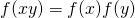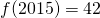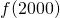# Functions Questions #2I am currently thinking of how to solve this following question, and will encourage any undergraduates to discuss this problem together. I’ll share a bit of what I’ve thought.

Ifis a bijective function that satisfiesand, what is the minimum value ofSo here the definition ofmeans thatis an automorphism of a free commutative monoid on an infinite generating set (of prime numbers). By the fundamental theorem of arithmetic, we know the set ofare a free commutative monoid over the set of prime numbers. It is a direct consequence of the general theory of monoids, thatsend primes to primes there.

Not readable? Change text.